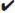# SAT Math Multiple Choice Question 829: Answer and Explanation

### Test Information

Question: 829

4. A graphic designer created an image for a school banner. He uses this image for smalland medium banners. To produce a large banner, the designer increases the size ofthe image by 112.5% to 18 inches tall by 22.5 inches wide. What was the size of theoriginal image?

• A. 15 inches by 17.5 inches
• B. 15 inches by 18 inches
• C. 16 inches by 18.5 inches
• D. 16 inches by 20 inches

Getting to the Answer: You're looking for dimensions that, when multiplied by 112.5% (or 1.125), the result is 18 inches by 22 inches. Start with A: 1.125 × 15 = 16.875 (not 18), so you can eliminate both A and B. Move on to C: 1.125 × 16 = 18, but 1.125 × 18.5 = 20.8125 (not 22.5), so (D) must be correct. (1.125 × 20 = 22.5)